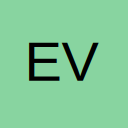# SUMIF Using Greater/Less Than Symbols In Text Criteria

• Hi,

I'm trying to get a simple Sumif formula to work. I have used this formula a hundred times before but this time the results are inaccurate. I suspect that it may be because of the math symbols which are used in the criteria text i.e. < and >

My formula is as follows:

=SUMIF('Raw Data Export'!D:D,"< No Project >",'Raw Data Export'!G:G)

A data extract from the sheet named "Raw Data Export" is attached.

Regards,

Leanne

## Files

• Re: Sumif A Cell Matches Text When The Text Contains Math Symbols

Hello,

Not sure why the math symbols are not recognized. How about as a work around, use the asterisk as a wildcard.

i.e
SUMIF( 'Raw Data Export'!D:D,"* No Project *",'Raw Data Export'!G:G)

HTH

JL

• Re: Sumif A Cell Matches Text When The Text Contains Math Symbols

The workaround works for me. Thanks, I didn't know wild cards could be used. It is weird why the original formula wouldn't work though.

• Re: Sumif A Cell Matches Text When The Text Contains Math Symbols

Quote

Not sure why the math symbols are not recognized

I reckon the < is interpreted as less than, as in [COLOR="Blue"]=COUNTIF(someRange, "<0")[/COLOR]. You'd think the tilde (~) would escape it, but it doesn't.

[SIZE=1]Entia non sunt multiplicanda sine necessitate.[/SIZE]

• Re: Sumif A Cell Matches Text When The Text Contains Math Symbols

Thought maybe this would work (found from an answer by SHG elsewhere), but it doesn't:
=SUMIF(D:D,CHAR(139) & " No Project " & CHAR(155),G:G)

SUMPRODUCT will work though:
=SUMPRODUCT(--(D2:D50="< No Project >"),G2:G50)

• Re: Sumif A Cell Matches Text When The Text Contains Math Symbols

Aha! [COLOR="Blue"]=SUMIF(D:D, "=< No Project >", G:G )[/COLOR]

[SIZE=1]Entia non sunt multiplicanda sine necessitate.[/SIZE]

• Re: SUMIF Using Greater/Less Than Symbols In Text Criteria

Personally, I would Replace all < * > with ( * ) to save further problems you may not even know about.

## Participate now!

Don’t have an account yet? Register yourself now and be a part of our community!